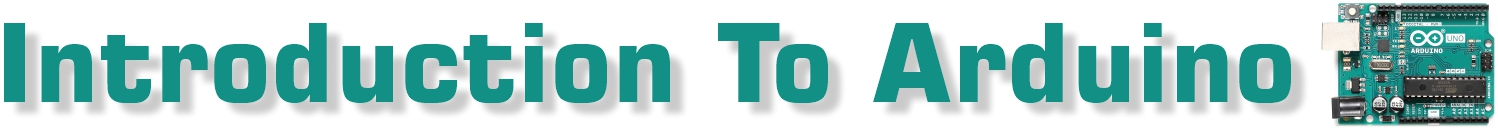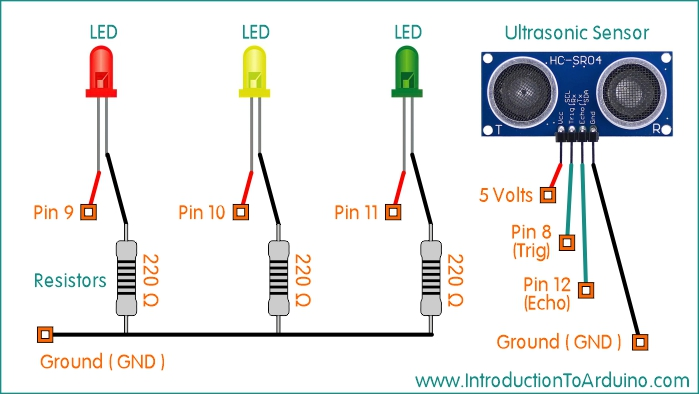Ultrasonic Distance Sensor and 3 LEDsArduino UNO R3 Pin Setup Overview: This project will allow you to use a HC-SR04 ultrasonic distance measuring sensor to determine the distance to an object and light different LEDs in set distance ranges. You will also be able to view the calculated distances in real time through the Serial Monitor. Parts List: 1 HC-SR04 Ultrasonic Sensor 3 LED 3 220 Ohm Resistor 1 Arduino UNO R3 1 Breadboard Connector Wires Code: ```//------------- Code Starts Here ---------------------------- //----------------------------------------- //Published by IntroductionToArduino.com //Created by Paul Illsley (www.paulillsley.com) //Please use and share so others can enjoy //----------------------------------------- // creating variables for the ultrasonic sensor data and assigning them to specific pins int trigger_pin = 8; int echo_pin = 12; // creating variables for distance calculations to be preformed later float duration; float cm; float inches; void setup() {  // set up serial monitor at a baud rate of 9600  Serial.begin (9600);  // declaring the pin modes as either output or input  pinMode(trigger_pin, OUTPUT);  pinMode(echo_pin, INPUT); } void loop() {  // activating the ultrasonic sensor to collect pulse length data  digitalWrite(trigger_pin, LOW);  delayMicroseconds(5);  digitalWrite(trigger_pin, HIGH);  delayMicroseconds(10);  digitalWrite(trigger_pin, LOW);  // placing the pulse length data in the "duration" variable  duration = pulseIn(echo_pin, HIGH);  // calculate the distance from the pulse length data  cm = (duration/2) / 29.1;  inches = (duration/2) / 74;  // Sending the raw and calculated distances (cm and inches) to the serial monitor  Serial.print("duration(ms):");  Serial.print(duration);  Serial.print(", cm:");  Serial.print(cm);  Serial.print(", inches:");  Serial.print(inches);  Serial.println();  // creating a statement saying:  // if the distance (cm) is greater than 30 then turn on pin 11 (LED #1)  // if the distance (cm) is greater than 15 and equal to or less than 30 then turn on pin 10 (LED #2)  // if the distance (cm) is anything else, turn on pin 9 (LED #3)  if(cm > 30.0)    {        digitalWrite(11,HIGH);        digitalWrite(10,LOW);        digitalWrite(9,LOW);    }    else if(cm > 15.0 && cm <= 30)    {        digitalWrite(11,LOW);        digitalWrite(10,HIGH);        digitalWrite(9,LOW);    }    else    {        digitalWrite(11,LOW);        digitalWrite(10,LOW);        digitalWrite(9,HIGH);    }       // delay 500 milliseconds (0.5 seconds)  delay(500); } //------------- Code Stops Here ---------------------------- ``` Created by Paul Illsley Return to www.introductiontoarduino.com